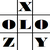anything in Excel...

It's Excel-o-lozy...

### "A+1=B", how to write this through formula in Excel?

If "3+1 = 4" then how to do "C+1=D" through a formula...

Simple...

=CHAR(CODE(B3)+1)

where cell "B3" can contain any single character and formula will result a next letter in series.

i.e. this formula will convert "a" or "A" in cell B3 as "b" or "B", respectively.

If "+1" in the formula is replaced with "+2" or "+3" then next 2nd or 3rd letter can be taken as result.

Cool??

www.Excelolozy.com -

Anything in Excel, be an Excelolozian...

for best MS Excel Tips visit http://www.Excelolozy.com

gotta query??? drop a mail at mail@excelolozy.com